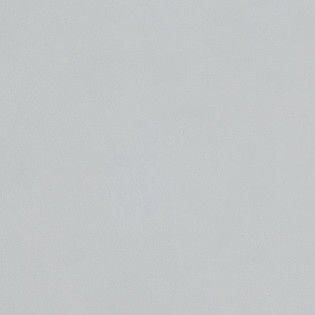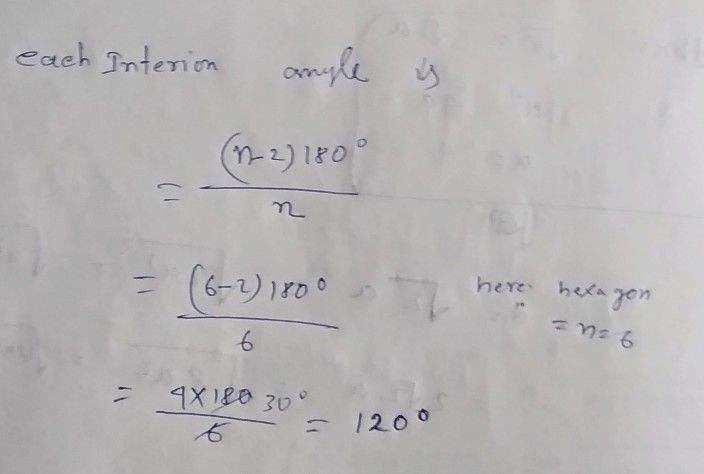Symbol
Problem$B$ The shape opposite is a regular hexagon. Jan $1$ $40$ "The hexagon is regular $40$ all the angles are the same, That makes each interior angle $\dfrac {360} {6}=60^{°}$ What mistakes has Jan made?
7th-9th grade
Other
SolutionQanda teacher - 1994SK